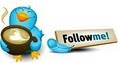#DAX Calculate Upper and Lower Control Limits• ### Question

•Hi guys,

I'd like to know if it is possible calculate upper and lower control limits in DAX for I use in charts.

I'm trying to do this calculate manually, the Average and Standard Deviation, with this i can calculate upper and lower limits.I created this example in Excel for showing what I want.Any idea for help me?

Thanks a lot!!!

Renato Freitas

Renato Freitas

Friday, December 21, 2012 12:26 PM

•Hi Jason,

I used the function SUMMARIZE together with AVERAGEX and STDEVX.P.

So, I got the results that I expected, Average and Standard Deviation by Month for calculate Lower and Upper Controls.

Measures

Average:=
CALCULATE(
(
AVERAGEX(
SUMMARIZE(
Sales
,Calendar[CalendarMonth]
,"Sales"
,CALCULATE(SUM(Sales[SalesAmount]))
)
,[Sales]
)
)
,ALLSELECTED(Sales)
)

Standard Deviation:=
CALCULATE(
(
STDEVX.P(
SUMMARIZE(
Sales
,Calendar[CalendarMonth]
,"Sales"
,CALCULATE(SUM(Sales[SalesAmount]))
)
,[Sales]
)
)
,ALLSELECTED(Sales)
)

Lower Control:=
CALCULATE([Average]-[Standard Deviation]*3,ALLSELECTED(Sales))

Upper Control:=
CALCULATE(([Average]+[Standard Deviation]*3),ALLSELECTED(sales))Renato Freitas

Wednesday, December 26, 2012 5:16 PM

### All replies

•You can calculate the mean (or average) and Standard deviation using builtin functions. But for upper and lower control limits, you must make your own calculations in dax. This is how I did it1) Create the mean measure

`Mean:=calculate(average(Table1[MeasureValue]), ALL(Table1))`

2) Create the Std Deviation measure.

`StdDev:=calculate(STDEV.P(Table1[MeasureValue]), ALL(Table1))`

3) Create UpperLimit measure

`UpperLimit:=[Mean] + 3 * [StdDev]`

4) Create lower limit measure.

`LowerLimit:=[Mean] - 3 *  [StdDev]`

Please note that I am passing the entire table as a filter. If you want the measures to change based on say Year, you might want to use the ALLExcept function and pass the columns on which the measures should be changed.

Cheers,
Jason | www.SqlJason.comMonday, December 24, 2012 8:02 PM
•Jason,

This built-in functions calculate the Average and Standard Deviation based on the all rows of the table.

My table has sales by day and I want to calculate the Average and Standard Deviation by Month. Is it possible?Thanks!!

Renato Freitas

Wednesday, December 26, 2012 3:25 PM
•Hi Jason,

I used the function SUMMARIZE together with AVERAGEX and STDEVX.P.

So, I got the results that I expected, Average and Standard Deviation by Month for calculate Lower and Upper Controls.

Measures

Average:=
CALCULATE(
(
AVERAGEX(
SUMMARIZE(
Sales
,Calendar[CalendarMonth]
,"Sales"
,CALCULATE(SUM(Sales[SalesAmount]))
)
,[Sales]
)
)
,ALLSELECTED(Sales)
)

Standard Deviation:=
CALCULATE(
(
STDEVX.P(
SUMMARIZE(
Sales
,Calendar[CalendarMonth]
,"Sales"
,CALCULATE(SUM(Sales[SalesAmount]))
)
,[Sales]
)
)
,ALLSELECTED(Sales)
)

Lower Control:=
CALCULATE([Average]-[Standard Deviation]*3,ALLSELECTED(Sales))

Upper Control:=
CALCULATE(([Average]+[Standard Deviation]*3),ALLSELECTED(sales))Renato Freitas

Wednesday, December 26, 2012 5:16 PM